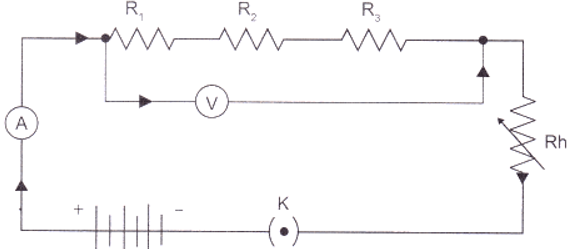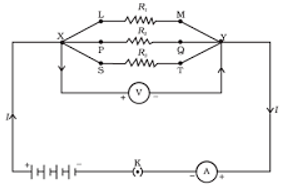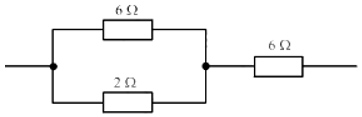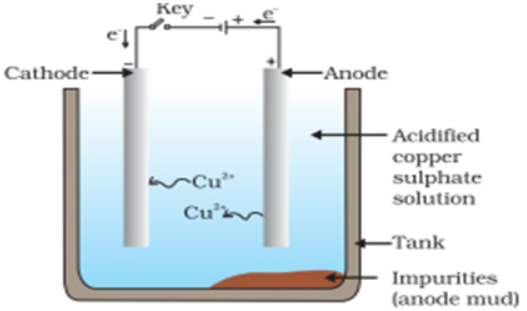# Case Study Questions Class 10 Science## myCBSEguide App

CBSE, NCERT, JEE Main, NEET-UG, NDA, Exam Papers, Question Bank, NCERT Solutions, Exemplars, Revision Notes, Free Videos, MCQ Tests & more.

• What are case study questions?
• Sample Papers with Case Study questions
• Class 10 Science Case Study question examples
• How to attempt the case-based questions in Science?## What are case study questions?

Questions based on case studies are some real-life examples. The questions are asked based on a given paragraph i.e. Case Study.  Usually, 4-5 questions are asked on the basis of the given passage. In most cases, these are either MCQs or assertion & reason type questions. Let’s take an example to understand. There is one paragraph on how nitrogen is generated in the atmosphere. On the basis of this paragraph, the board asks a few objective-type questions. In other words, it is very similar to the unseen passages given in language papers. But the real cases may be different. So, read this article till the end to understand it thoroughly.

### What is CBE?

CBSE stands for competency-based education. The case study questions are part of this CBE. The purpose of CBE is to demonstrate the learning outcomes and attain proficiency in particular competencies.

### Questions on Real-life Situations

As discussed the case study questions are based on real-life situations. Especially for grade 10 science, it is very essential to have the practical knowledge to solve such questions. Here on the myCBSEguide app, we have given many such case study paragraphs that are directly related to real-life implications of the knowledge.

## Sample Papers with Case Study Questions

Class 10 Science Sample Papers with case study questions are available in the myCBSEguide App. There are 4 such questions (Q.No.17 to 20) in the CBSE model question paper. If you analyze the format, you will find that the MCQs are very easy to answer. So, we suggest you, read the given paragraph carefully and then start answering the questions. In some cases, you will find that the question is not asked directly from the passage but is based on the concept that is discussed there. That’s why it is very much important to understand the background of the case study paragraph.

### CBSE Case Study Sample Papers

You can download CBSE case study sample papers from the myCBSEguide App or Student Dashboard. Here is the direct link to access it.

### Case Study Question Bank

As we mentioned that case study questions are coming in your exams for the last few years. You can get them in all previous year question papers issued by CBSE for class 1o Science. Here is the direct link to get them too.

## Class 10 Science Case Study Question Examples

As you have already gone through the four questions provided in the CBSE model question paper, we are proving you with other examples of the case-based questions in the CBSE class 10 Science. If you wish to get similar questions, you can download the myCBSEguide App and access the Sample question papers with case study-type questions.

### Case-based Question -1

Salt of a strong acid and strong base is neutral with a pH value of 7. NaCl common salt is formed by a combination of hydrochloride and sodium hydroxide solution. This is the salt that is used in food. Some salt is called rock salt bed of rack salt was formed when seas of bygone ages dried up. The common salt thus obtained is an important raw material for various materials of daily use, such as sodium hydroxide, baking soda, washing soda, and bleaching powder.

1. Which of the following does not form an acidic salt?
1. Phosphoric acid
2. Carbonic acid
3. Hydrochloric acid
4. Sulphuric acid
2. Which of the following salts has no water of crystallization?
1. Blue vitriol
2. Washing soda
3. Baking soda
4. Gypsum
3. The formula of baking soda is
1. K2CO
2. KHCO3
3. NaHCO3
4. Na2CO3
4. Which of the following is treated with chlorine to obtain bleaching powder
1. CaSO4
2. Ca(OH)2
3. Mg(OH)2
4. KOH
5. Which of the following salt is used for removing the permanent hardness of water
1. Washing soda
2. Baking soda
3. Bleaching powder
4. NaOH

### Case-based Question -2

In resistance for a system of the resistor, there are two methods of joining the resistors together as shown belowIt showed an electric current in which 3 resistors having resistors R1, R2 and R3 respectively are joined end to end i.e series. While the combination of the resistor in which 3 resistors are connected together which points X and Y are said to be parallel.

1. The total potential difference across a combination of a resistor in series is equal to
1. V1 + V2 + V3
2. V– V+V2
3. V+ V2
4. None of these
2. In a series combination of resistors, the current is
1. same at every point of the circuit
2. different at every point of the circuit
3. zero
4. can not be determined
3. The electrical energy disputed in the resistor is given by
1. W = VIT
2. W = VIR
3. W = RIT
4. W = RT
4. If 5 resistors, each of value 0.2 ohm are connected in series what will be the resultant resistance
1. 1 ohm
2. 10 ohm
3. 6 ohm
4. 8 ohm
5. The figure given below shows three resistors.Their combined resistance is:

1. 16 ΩΩ
2. 14 ΩΩ
3. 203Ω203Ω
4. 152Ω152Ω

### Case-based Question -3

In the electrolytic refining of copper. The electrolyte is a solution of acidified copper sulphate. There are an anode and cathode. Refining is carried out by passing an electric current.1. The anode is ________.
1. pure strips
2. impure copper
3. refined copper
4. none of these
2. Anode mud consists of:
1. insoluble impurities
2. soluble impurities
3. pure metal
4. impure metal
3. Which of the following are refined electrolytically: (A) Au, (B) Cu, (C) Zn, (D) K
1. A and B
2. B and C
3. A, B and C
4. B, C and D
4. On passing, electric current Cu is deposited on:
1. cathode
2. anode
3. bottom of cathode
4. bottom of anode
5. Which one of the following four metals would be displaced from the solution of its salt by the other three metals?
1. Zn
2. Mg
3. Cu
4. Ag

## How to Attempt the Case-Based Questions in Science?

Before answering this question, let’s read the text given in question number 17 of the CBSE Model Question Paper.

All living cells require energy for various activities. This energy is available by the breakdown of simple carbohydrates either using oxygen or without using oxygen.

See, there are only two sentences and CBSE is asking you 5 questions based on these two sentences. Now let’s check the first questions given there.

Energy in the case of higher plants and animals is obtained by
a) Breathing
b) Tissue respiration
c) Organ respiration
d) Digestion of food

Now let us know if you can relate the question to the paragraph directly. The two sentences are about energy and how it is obtained. But neither the question nor the options have any similar text in the paragraph.

So the conclusion is, in most cases, you will not get direct answers from the passage. You will get only an idea about the concept. If you know it, you can answer it but reading the paragraph even 100 times is not going to help you.Test Generator

Create question papers online with solution using our databank of 5,00,000+ questions and download as PDF with your own name & logo in minutes.